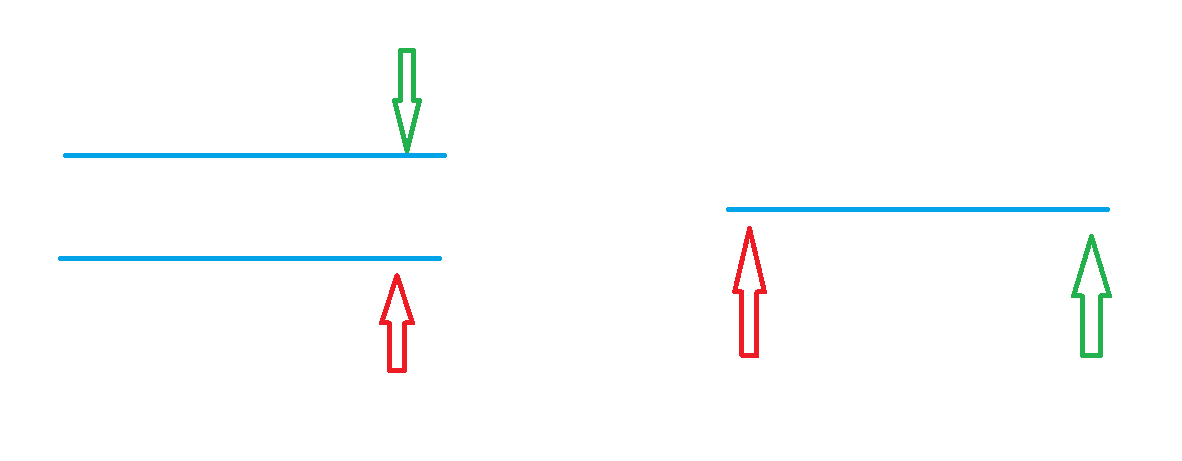# 双指针算法和位运算 & 离散化和区间合并### 双指针算法image.png

for(int i=0,j=0;i<n;i++){    while(j<i&&check(i,j))j++;    //具体问题的逻辑}    //常见问题分类：        1. 对于一个序列，用两个指针维护一段区间。        2. 对于两个序列，维护某种次序，比如归并排序中合并两个有序序列的操作

#include<iostream>#include<cstring>#include<cstdio>using namespace std;int main(){    char str;    //for(int i=0;i<1000;i++)cin>>str[i];    fgets(str,1000,stdin);    int n=strlen(str);    for(int i=0;i<n;i++){        int j=i;        while(j<n&&str[j]!=' ')j++;                //这道问题的具体逻辑        for(int k=i;k<j;k++)cout<<str[k];        cout<<endl;                i=j;    }    return 0;}

#### 最长连续不重复子序列image.png

j 为绿色，i 为蓝色

1. 遍历数组 a 中的每一个元素 a[i], 对于每一个 i，找到 j 使得双指针[j, i]维护的是以 a[i]结尾的最长连续不重复子序列，长度为 i - j + 1, 将这一长度与 r 的较大者更新给 r。

2. 对于每一个 i，如何确定 j 的位置：由于[j, i - 1]是前一步得到的最长连续不重复子序列，所以如果[j, i]中有重复元素，一定是 a[i]，因此右移 j 直到 a[i]不重复为止（由于[j, i - 1]已经是前一步的最优解，此时 j 只可能右移以剔除重复元素 a[i]，不可能左移增加元素，因此，j 具有“单调性”、本题可用双指针降低复杂度）。

3. 用数组 s 记录子序列 a[j ~ i]中各元素出现次数，遍历过程中对于每一个 i 有四步操作：cin 元素 a[i] -> 将 a[i]出现次数 s[a[i]]加 1 -> 若 a[i]重复则右移 j（s[a[j]]要减 1） -> 确定 j 及更新当前长度 i - j + 1 给 r。

#include<iostream>using namespace std;const int N = 100010;int n;int a[N],s[N];int main(){    cin>>n;    for(int i=0;i<n;i++)cin>>a[i];    int res=0;    for(int i=0,j=0;i<n;i++){        s[a[i]]++;        while(s[a[i]]>1){            s[a[j]]--;            j++;        }        res=max(res,i-j+1);    }    cout<<res<<endl;    return 0;}

### 位运算

#include<iostream>using namespace std;int main(){    int n=10;//将十转换成二进制    for(int k=3;k>=0;k--)cout<<(n>>k&1);    return 0;}

x&-x==x&(~x+1)

#### 二进制中 1 的个数

#include<iostream>using namespace std;int lowbit(int x){    return x&-x;}int main(){    int n;    cin>>n;    while(n--){        int x;        cin>>x;        int res=0;        while(x)x-=lowbit(x),res++;//每次减去最后一位1                cout<<res<<' ';    }    return 0;}

### 离散化（整数离散化）

vector<int> alls;//存储所有待离散化的值

sort(alls.begin(),alls.end());//将所有的值排序

alls.erase(unique(alls.begin(),alls.end()),alls.end());//去掉重复元素

int find(int x){    int l=0,r=alls.size()-1;    while(l<r){        int mid=l+r>>1;        if(alls[mid]>=x)r=mid;        else l=mid+1;    }    return r+1;}

#### 区间和

#include <iostream>#include <vector>#include <algorithm>using namespace std;typedef pair<int, int> PII;const int N = 300010;int a[N], s[N];int n, m;vector<int> alls;vector<PII> add, query;int find(int x){    int l = 0, r = alls.size() - 1;    while(l < r)    {        int mid = l + r >> 1;        if(alls[mid] >= x) r = mid;        else l = mid + 1;    }    return r + 1;}vector<int>:: iterator unique(vector<int> &a){    int j = 0;    for(int i = 0; i < a.size(); i ++)        if(!i || a[i] != a[i - 1])            a[j ++ ] = a[i];    return a.begin() + j;}int main(){    cin >> n >> m;    for(int i = 0; i < n; i ++ )    {        int x, c;        cin >> x >> c;        add.push_back({x, c});        alls.push_back(x);    }    for(int i = 0; i < m; i ++ )    {        int l, r;        cin >> l >> r;        query.push_back({l, r});        alls.push_back(l);        alls.push_back(r);    }    sort(alls.begin(), alls.end());    alls.erase(unique(alls), alls.end());    for(auto item : add)    {        int x = find(item.first);        a[x] += item.second;    }    for(int i = 1; i <= alls.size(); i ++ ) s[i] = s[i - 1] + a[i];    for(auto item : query)    {        int l = find(item.first), r = find(item.second);        cout << s[r] - s[l - 1] << endl;    }    return 0;}

### 区间合并

#include <iostream>#include <vector>#include <algorithm>using namespace std ;typedef pair<int,int> pii ;vector<pii>nums,res ;int main(){    int st=-2e9,ed=-2e9 ;                           //ed代表区间结尾，st代表区间开头    int n ;    scanf("%d",&n) ;     while(n--)    {        int l,r ;         scanf("%d%d",&l,&r) ;        nums.push_back({l,r}) ;    }    sort(nums.begin(),nums.end()) ;                 //按左端点排序    for(auto num:nums)                       {        if(ed<num.first)                            //情况1：两个区间无法合并        {            if(ed!=-2e9) res.push_back({st,ed}) ;   //区间1放进res数组            st=num.first,ed=num.second ;            //维护区间2        }        //情况2：两个区间可以合并，且区间1不包含区间2，区间2不包含区间1        else if(ed<num.second)              ed=num.second ;                         //区间合并    }      //(实际上也有情况3：区间1包含区间2，此时不需要任何操作，可以省略)    //注：排过序之后，不可能有区间2包含区间1    if(st!=-2e9&&ed!=-2e9) res.push_back({st,ed});    //剩下还有一个序列，但循环中没有放进res数组，因为它是序列中的最后一个序列    /*    for(auto r:res)        printf("%d %d\n",r.first,r.second) ;    puts("") ;    */    //(把上面的注释去掉，可以在调试时用)    printf("%d",res.size()) ;           //输出答案    return 0 ;}## 评论